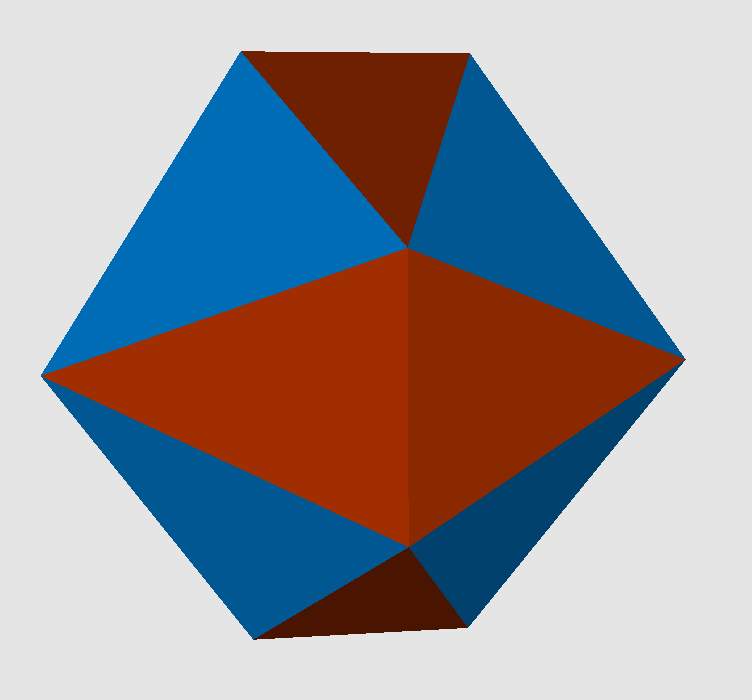Acronym ... Name cubically-diminished dodecahedron,bi-tetrahedrally-diminished dodecahedron `` Circumradius sqrt[(9+3 sqrt(5))/8] = 1.401259 Vertex figure [3,t,3,T,T] Dihedral angles (at margins) between {3} and {(t,T,T)}:   arccos(-sqrt[(5+2 sqrt(5))/15]) = 142.622632° between {(t,T,T)} and {(t,T,T)}:   arccos(-1/sqrt(5)) = 116.565051° Confer cube   doe   tet   tet-dim-doe   general pyritohedral ike

The non-regular (ffx)-triangles {(t,T,T)} are faceted regular pentagons. Its vertex angles are t = 36° resp. T = 72°. Their longer side tT is scaled by the golden ratio f = (1+sqrt(5))/2 = 1.618034. The regular triangles use the f-scaled edges only.

This polyhedron can be seen as a special pyritohedral symmetric variant of ike. The according more general variant is oca cao aoc&#zd, then with d = sqrt[(a2-ac+c2)/2].

Incidence matrix according to Dynkin symbol

```oxF xFo Fox&#zf   (F=ff) → heights = 0
(tegum sum of 3 mutually perp. (x,F)-{4})

o.. o.. o..    & | 12 | 1  4 |  3 2
-----------------+----+------+-----
... x.. ...    & |  2 | 6  * |  2 0  x-edges
oo. oo. oo.&#f & |  2 | * 24 |  1 1  f-edges
-----------------+----+------+-----
ox. ... ...&#f & |  3 | 1  2 | 12 *  {(t,T,T)}
ooo ooo ooo&#f   |  3 | 0  3 |  * 8  {3}
```